This is just a post with some thoughts on how I am planning to go about setting up the action space for the Big 2 reinforcement learner I am working on at the moment. For anyone unfamiliar with the game you can see the rules and try out a single player version of the game against a very basic AI (essentially just a series of "if" statements) here. The plan for this project is to train a neural network that learns to play the game through self-play reinforcement learning. There are a couple of things that make this an interesting and challenging game to apply reinforcement learning to (in my opinion anyway!). Firstly, unlike games such as Chess and Go it's a game of imperfect information, i.e. each player does not have full knowledge of the current state of the game because they do not know what cards the other players have (although of course things can be deduced as the game progresses). Secondly, Big 2 is most naturally a four player game whereas the most famous applications of reinforcement learning have been primarily to two player games and finally (and in this case similarly to Chess) the state of possible actions that can be taken is actually fairly complicated and state dependent. This is because the actions that can be taken depend on what the previous player has played and whether or not you have "control" of the game, and include all 2,3,4 and 5 card "poker hands" i.e. pairs, three of a kinds, two pairs, four of a kinds, straights, flushes, full houses and straight flushes. This post is concerned with how I am planning to go about representing all of the actions that the neural network has available to it in a sensible way such that it is able to learn a sensible policy.

The reason this is tricky is because of being able to play hands with more than one card in them as it is possible for there to be many ways to do this. Let us consider the most extreme example where we have the following starting hand made up of 13 cards of the same suit: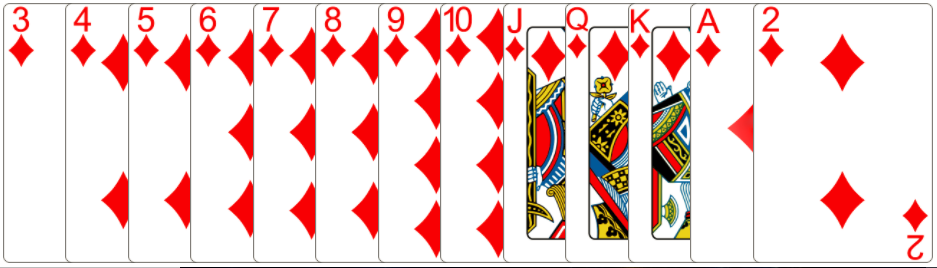Imagine that the previous player has played a straight. Clearly we can play a flush that beats this, but which flush should be chosen? In this case we can choose any of the 5 cards to make a flush which means there are $${13 \choose 5} = 1287$$ unique flushes that can be played here. I will do a separate post about what information will be used as the input state to the neural network but I think at the moment that it will most likely include the following (along with other information such as cards other people have played so far):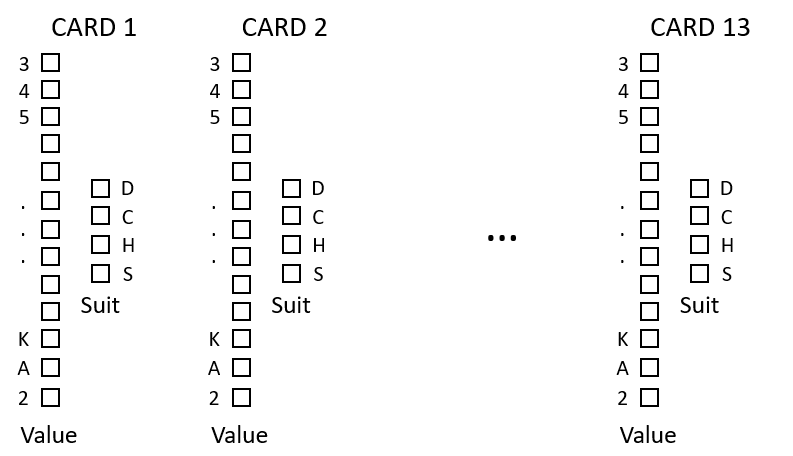i.e. for each of the 13 initial cards there will be 13 binary values (0 or 1) for the value of each card as well as for the suit. The alternative would be to use 52 binary inputs representing whether each card is present in the current hand but I think the first method will be necessary if we're going to use a neural network that can choose any possible action as the can use the indices of the cards (1-13) to systematically describe all of the available actions. e.g. we can say that $$\{1,3,4,6,10 \}$$ would be the 5 card hand represented by the 1st, 3rd, 4th, 6th and 10th card in the current hand (of course this may not be a real hand, in which case the move would not be valid). If we are to consider every possible action we must allow for $${13 \choose 5}=1287$$ five card moves, $${13 \choose 4}=715$$ four card moves, $${13 \choose 3}=286$$ three card moves, $${13 \choose 2} = 78$$ two card moves and 13 one card moves. In fact this is actually more than is strictly necessary as for 2,3 and 4 card hands there are certain index combinations that will never be possible (e.g. card 1 and card 13 can never be played together as a pair assuming the hand is sorted initially. But it might be better to not actually order the cards - this might need some more thought!) This leaves a total of 2379 possible actions (theoretically, most will of course not correspond to valid moves at any particular time). Initially I was worried that this would be far too many actions for a neural network to output and to be honest I am still worried, but reading the recent paper where the Alpha Go algorithm was applied to Chess I read the following: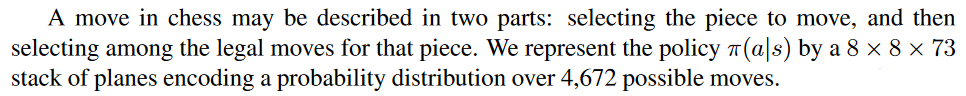So clearly their neural network considered an output space with an even larger number of possible moves, but they also have a hell of a lot more computing power at their disposal! Still this gives me hope that this approach is at least worth a go and may be able to work!

To get anywhere with this approach we need a way of indexing the actions for a particular combination of cards so that we do not have to consider whether every single one of the theoretically possible actions are available each time a hand is updated. So for 5 card hands we can define an action by a set of integers$$\{c_1, c_2, c_3, c_4, c_5 \}$$ (with $$c_5 > c_4 > c_3 > c_2 > c_1$$ ) which we need to convert into a unique integer between 1 and 1287. It took me probably longer than it should have done to come up with a way to do this, but we can evaluate the sum:

$$\begin{eqnarray}a(c_1,c_2,c_3,c_4,c_5) = \sum_{i'=1}^{c_1-1} \sum_{j'=i'+1}^{10} \sum_{k'=j'+1}^{11} \sum_{l'=k'+1}^{12} \sum_{m'=l'+1}^{13} 1 + \sum_{i'=c_1+1}^{c_2-1} \sum_{j'=i'+1}^{11} \sum_{k'=j'+1}^{12} \sum_{l'=k'+1}^{13} 1 \nonumber \\ + \sum_{i'=c_2+1}^{c_3-1} \sum_{j'=i'+1}^{12} \sum_{k'=j'+1}^{13} 1 + \sum_{i'=c_3+1}^{c_4-1} \sum_{j'+1}^{13} 1 + (c_5-c_4) \end{eqnarray}$$

which can be evaluated with Mathematica: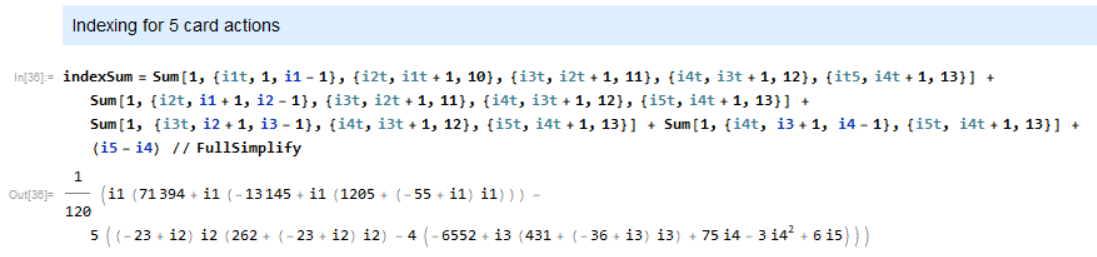Now that I think about it we need to be able to invert this as well, i.e. for a given action index retrieve the $$\{c_1, c_2, c_3, c_4, c_5 \}$$ indices. This calculation can be done once and we can just store the result in memory so should be trivial.

The reason this is useful is that it means we don't have to evaluate all 1287 combinations of $$\{c_i\}$$ and check whether each is a valid move in the current state. Imagine we have the following hand and are trying to calculate the 5 card options available to us: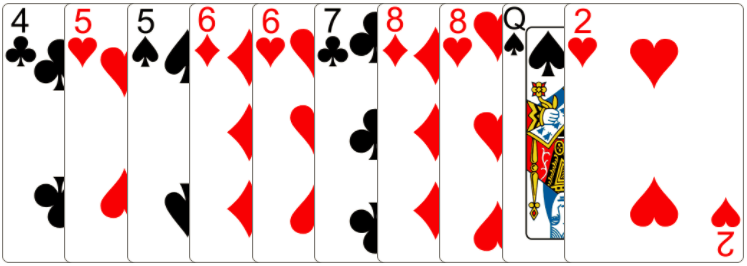we can play a straight with any combination of 4,5,6,7,8 (one of each). In general for calculating the straights available we can calculate the set of consecutive numbers (including repeats), so in the example above this would be $$\{4C,5H,5S,6D,6H,7C,8D,8H,QS,2H \}$$ and then calculate of the actions which are actually valid in this state and so that need to be considered by the neural network. We can do similar things for flushes and full houses as well as for the 4,3,2 card hands.

This is the approach I would really like to take as this is basically not giving the neural network any human knowledge about the game and letting it learn everything from scratch which to me would be a lot more elegant and interesting. The other approach I have been considering is to consider a much smaller action space that uses a bit more game knowledge with options that represent what I consider to be reasonable potential options from any given state. E.g: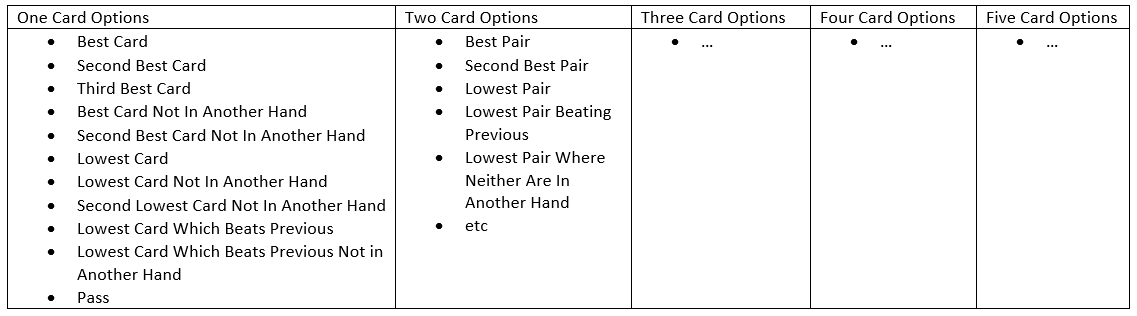This approach requires a lot more calculation and analyzing each hand the neural network sees to find out e.g. what the best card is that is not apart of another hand. This quickly becomes inelegant and pretty annoying to code up and also means that there are possible moves that the neural network will never consider in certain situations. Given that I'm not an expert player at all I would rather only fall back on this approach if it seems like the action space is too large with the first approach I have suggested.

EDIT

Having thought about it a bit more I have a much better solution I think which slightly reduces the size of the action space which needs to be considered by ensuring that we use a sorted hand and then just use a lookup table - for example considering 5 card moves we define a look up matrix of dimensions $$13 \times 13 \times 13 \times 13 \times 13$$ which we can index with the values of $$\{c_i \}$$. Although most entries will correspond to moves which can never be made $$13^5 = 371293$$ is small enough that it doesn't take up too much memory. The following code is used to create a lookup table and an inverse lookup table:

nActions5 = 1287 #number of potentially possible 5 card moves
fiveCardIndices = np.zeros((13,13,13,13,13),dtype=int)
inverseFiveCardIndices = np.zeros((nActions5,5),dtype=int)
c = 0
for c1 in range(9):
for c2 in range(c1+1,10):
for c3 in range(c2+1,11):
for c4 in range(c3+1,12):
for c5 in range(c4+1,13):
fiveCardIndices[c1][c2][c3][c4][c5] = c
inverseFiveCardIndices[c] = c1
inverseFiveCardIndices[c] = c2
inverseFiveCardIndices[c] = c3
inverseFiveCardIndices[c] = c4
inverseFiveCardIndices[c] = c5
c += 1

For four card hands (i.e. two pairs or four of a kinds) we really don't need to consider all $${13 \choose 4} = 715$$ moves as many are not allowable in any situation. Instead we can just create a lookup table as follows:

nActions4 = 330
fourCardIndices = np.zeros((13,13,13,13), dtype=int)
inverseFourCardIndices = np.zeros((nActions4,4), dtype=int)
c=0
for c1 in range(10):
nextInd = np.min([c1+3,10])
for c2 in range(c1+1,nextInd+1):
for c3 in range(c2+1,12):
nextInd = np.min([c3+3,12])
for c4 in range(c3+1,nextInd+1):
fourCardIndices[c1][c2][c3][c4] = c
inverseFourCardIndices[c] = c1
inverseFourCardIndices[c] = c2
inverseFourCardIndices[c] = c3
inverseFourCardIndices[c] = c4
c += 1

And we can do a similar thing for three and two card hands as well. Doing this we have a total number of actions of $$13 + 33 + 31 + 330 + 1287 = 1694$$ rather than 2379 moves from the previous method. This possibly doesn't help that much because we still have an enormous number of possible 5 card moves but every little helps!